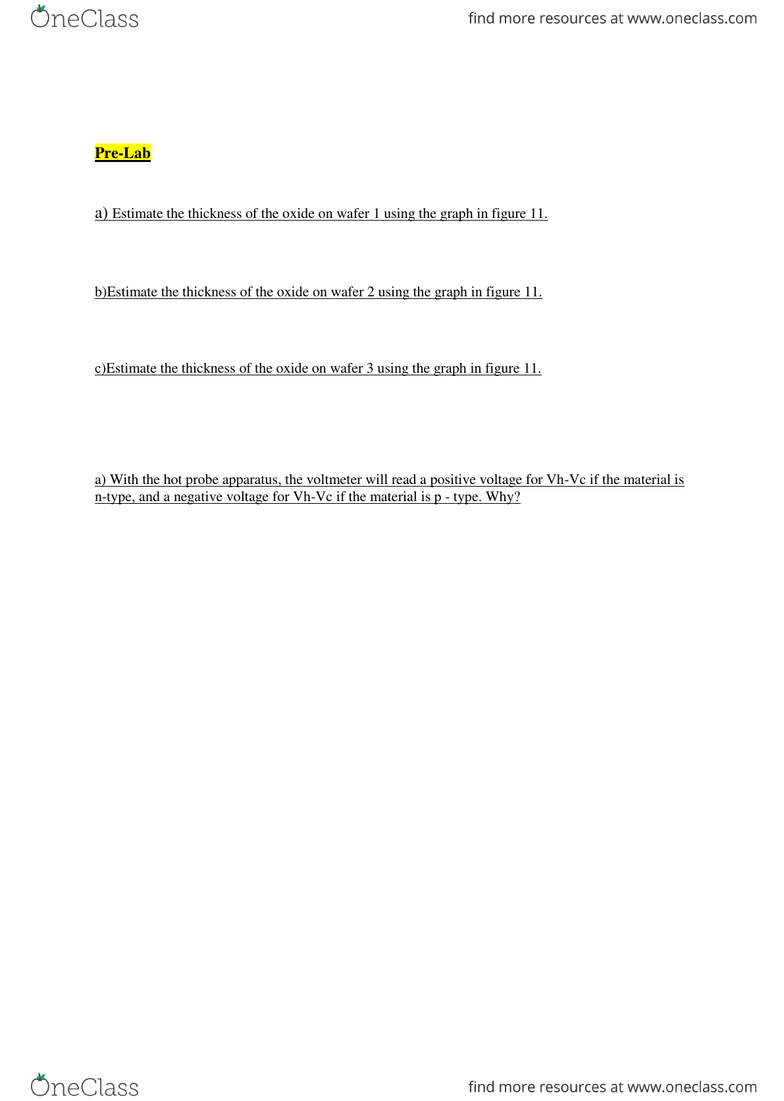Class Notes (1,000,000)
CA (620,000)
UW (20,000)
ECE (200)
ECE331 (5)
Lecture 1

ECE331 Lecture Notes - Lecture 1: He Zi, Charge Carrier, Voltmeter

Department
Electrical and Computer Engineering
Course Code
ECE331
Professor
C.R.Selvakumar
Lecture
1

Page:
of 1ECE 331 Lab 1 Report
By Wei, He / Zi, Li
Pre-Lab
4.4 Estimation of Oxide Thickness
a) Estimate the thickness of the oxide on wafer 1 using the graph in figure 11.
Wafer 1 has wet growth at 1100 degrees Celsius and 50 minutes, judging by figure 11, its thickness of
oxide is approximately 0.65µm.
b)Estimate the thickness of the oxide on wafer 2 using the graph in figure 11.
Wafer 2 has wet growth at 1100 degrees Celsius and 36 minutes, judging by figure 11, its thickness of
oxide is approximately 0.5μm.
c)Estimate the thickness of the oxide on wafer 3 using the graph in figure 11.
Since wafer 3 has the combined oxide formed from step 1 and 4, its thickness is approximately the
sum of oxide formed in step 1 and 4. Thus its thickness is approximately to be 1.15μm.
4.5 Carrier Type by Hot Probe
a) With the hot probe apparatus, the voltmeter will read a positive voltage for Vh-Vc if the material is
n-type, and a negative voltage for Vh-Vc if the material is p - type. Why?
In n-type material, each impurity atom donates an electron, and thereby becomes a positively charged
ion; Electrons are majority carriers and when point h is heated, electron flows from point h to c, what
is left out in point h is holes, which has a positive polarity. Thus the voltage at point h should be
bigger than point c, Vh - Vc is a positive value. In p-type material, the majority carrier is holes.
Holes flows from point h to c, what is left at h is electrons, which has a negative polarity. Thus
voltage at h is smaller than c, the voltmeter shows a negative value for Vh - Vc.